Electron. J. Differential Equations, Vol. 2022 (2022), No. 25, pp. 1-29.

### Fractional Kirchhoff Hardy problems with weighted Choquard and singular nonlinearity Sarika Goyal, Tarun Sharma

Abstract:
In this article, we study the existence and multiplicity of solutions to the fractional Kirchhoff Hardy problem involving weighted Choquard and singular nonlinearity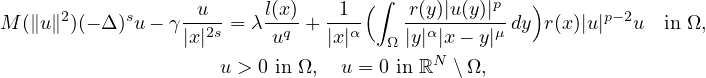where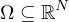is an open bounded domain with smooth boundary containing 0 in its interior,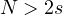with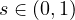,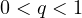,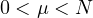,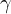and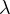are positive parameters,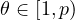with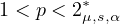, where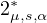is the upper critical exponent in the sense of weighted Hardy-Littlewood-Sobolev inequality. Moreover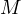models a Kirchhoff coefficient, l is a positive weight and r is a sign-changing function. Under the suitable assumption on l and r, we established the existence of two positive solutions to the above problem by Nehari-manifold and fibering map analysis with respect to the parameters.The results obtained here are new even for s=1.

Submitted December 30, 2021. Published March 25, 2022.
Math Subject Classifications: 35A15, 35J75, 36B38.
Key Words: Fractional Kirchhoff Hardy operator; singular nonlinearity; \hfill\break\indent weighted Choquard type nonlinearity; Nehari-manifold; fibering map.

Show me the PDF file (461 KB), TEX file for this article.Tarun Sharma Department of Mathematics Bennett University Greater Noida, Uttar Pradesh, India email: tarunsharma80065@gmail.com Sarika Goyal Department of Mathematics Bennett University Greater Noida, Uttar Pradesh, India email: sarika1.iitd@gmail.com